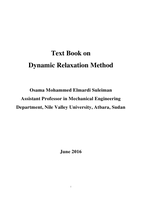اسم الكتاب

# Text Book on Dynamic Relaxation Method

القسم

ركن الهندسة الميكانيكية - Mechanical Engineering

عن الكتاب

The method of dynamic relaxation in its early stages of development was perceived as a numerical finite difference technique. It was first used to analyze structures, then skeletal and cable structures, and plates. The method relies on a discretized continuum in which the mass of the structure is assumed to be concentrated at given points (i.e. nodes) on the surface. The system of concentrated masses oscillates about the equilibrium position under the influence of out of balance forces. With time, it comes to rest under the influence of damping. The iterative scheme reflects a process, in which static equilibrium of the system is achieved by simulating a pseudo dynamic process in time. In its original form, the method makes use of inertia term, damping term and time increment.
Chapter one includes introduction to dynamic relaxation method (DR) which is combined with finite differences method (FD) for the sake of solving ordinary and partial differential equations, as a single equation or as a group of differential equations. In this chapter the dynamic relaxation equations are transformed to artificial dynamic space by adding damping and inertia effects. These are then expressed in finite difference form and the solution is obtained through iterations.
In chapter two the procedural steps in solving differential equations using DR method were applied to the system of differential equations (i.e. ordinary and/ or partial differential equations). The DR program performs the following operations: Reads data file; computes fictitious densities; computes velocities and displacements; checks stability of numerical computations; checks convergence of solution; and checks wrong convergence. At the end of this chapter the dynamic relaxation (DR) numerical method coupled with the finite differences discretization technique is used to solve nonlinear ordinary and partial differential equations. Subsequently, a FORTRAN program is developed to generate the numerical results as analytical and/ or exact solutions.
Chapter three discusses the importance of using numerical methods in solving differential equations and stresses the use of dynamic relaxation technique in these applications.
The book is suitable as a textbook for a first course on dynamic relaxation technique in civil and mechanical engineering curricula. It can be used as a reference by engineers and scientists working in industry and academic institutions.

Author
Assistant Professor
Osama Mohammed Elmardi Suleiman

صاحب الكتاب

عدد القراء

1404

عدد مرات التحميل

33

التقييم

تاريخ الأضافة

2018-05-208:33 PM

نوع الملف

application / pdf

حجم الكتاب

0.71 ميجا بايت

التعليقات

{{ comment.created_at }}

{{ whoCommented(comment) }}

{{ comment.comment }}